## Polar Form

The polar form of a complex number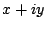is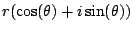where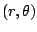are any choice of polar coordinates that represent the point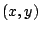in rectangular coordinates. Recall that you can find the polar form of a point using that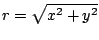and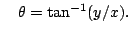NOTE: The existence'' of complex numbers wasn't generally accepted until people got used to a geometric interpretation of them.

Example 4.3.2   Find the polar form of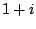.
Solution. We have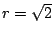, so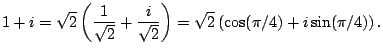Example 4.3.3   Find the polar form of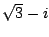.
Solution. We have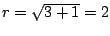, so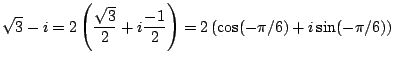[[A picture is useful here.]]

Finding the polar form of a complex number is exactly the same problem as finding polar coordinates of a point in rectangular coordinates. The only hard part is figuring out what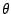is.

If we write complex numbers in rectangular form, their sum is easy to compute: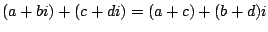The beauty of polar coordinates is that if we write two complex numbers in polar form, then their product is very easy to compute: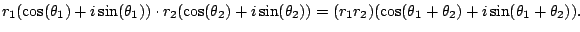The magnitudes multiply and the angles add. The above formula is true because of the double angle identities for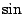and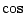(and it is how I remember those formulas!).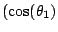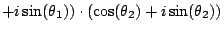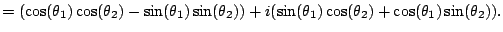For example, the power of a singular complex number in polar form is easy to compute; just power the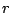and multiply the angle.

Theorem 4.3.4 (De Moivre's)   For any integer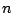we have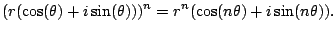Example 4.3.5   Compute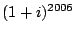.
Solution. We have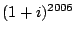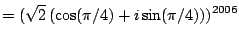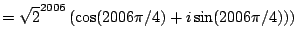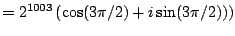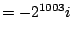To get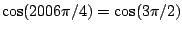we use that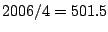, so by periodicity of cosine, we have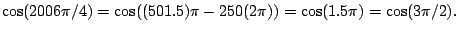EXAM 1: Wednesday 7:00-7:50pm in Pepper Canyon 109 (!) Today: Supplement 1 (get online; also homework online) Wednesday: Review Bulletin board, online chat, directory, etc. - see main course website. Review day - I will prepare no LECTURE; instead I will answer questions. Your job is to have your most urgent questions ready to go! Office hours moved: NOT Tue 11-1 (since nobody ever comes then and I'll be at a conference); instead I'll be in my office to answer questions WED 1:30-4pm, and after class on WED too. Office: AP&M 5111

 Quick review: Given a pointin the plane, we can also view it asor in polar form as. Polar form is great since it's good for multiplication, powering, and for extracting roots: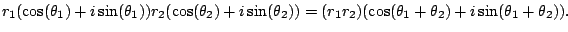(If you divide, you subtract the angle.) The point is that the polar form works better with multiplication than the rectangular form. Theorem 4.3.6 (De Moivre's)   For any integerwe haveSince we know how to raise a complex number in polar form to theth power, we can find all numbers with a given power, hence find theth roots of a complex number.

Proposition 4.3.7 (th roots)   A complex number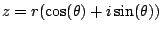hasdistinctth roots: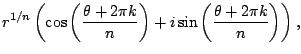for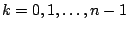. Here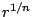is the real positive-th root of.

As a double-check, note that by De Moivre, each number listed in the proposition hasth power equal to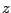.

An application of De Moivre is to computing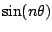and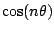in terms of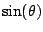and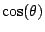. For example,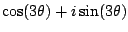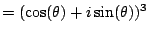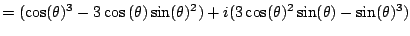Equate real and imaginary parts to get formulas for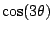and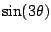. In the next section we will discuss going in the other direction, i.e., writing powers ofandin terms ofand cosine.

Example 4.3.8   Find the cube roots of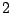.
Solution. Writein polar form as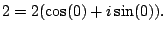Then the three cube roots ofare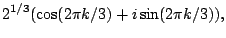for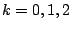. I.e.,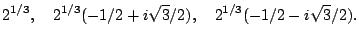William Stein 2006-03-15